# Test: Homogeneous Differential Equations

## 10 Questions MCQ Test Mathematics (Maths) Class 12 | Test: Homogeneous Differential Equations

Description
This mock test of Test: Homogeneous Differential Equations for JEE helps you for every JEE entrance exam. This contains 10 Multiple Choice Questions for JEE Test: Homogeneous Differential Equations (mcq) to study with solutions a complete question bank. The solved questions answers in this Test: Homogeneous Differential Equations quiz give you a good mix of easy questions and tough questions. JEE students definitely take this Test: Homogeneous Differential Equations exercise for a better result in the exam. You can find other Test: Homogeneous Differential Equations extra questions, long questions & short questions for JEE on EduRev as well by searching above.
QUESTION: 1

### The solution of the differential equation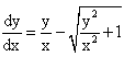is :

Solution:

dy/dx = y/x - (y2/x2 + 1)½…………………(1)
the equation is homogenous,so y = Vx
dy/dx = V + xdv/dx
Put the value of dy/dx in eq(1)
V + xdv/dx = V - (V2 + 1)½
= ∫-dv(v2 + 1)½ = ∫dx/x
= log|v + (v2 + 1)½| = -logx + logc
= log|y/x + ((y/x)2 + 1)½| =  log c/x
=|y/x + ((y/x)2 + 1)½| =  c/x

QUESTION: 2

### The solution of the differential equationis :

Solution:

dy/dx = (x2 + 3y2)/2xy………….(1)
Let y = vx
dy/dx = v + xdv/dx
Substitute the value of y and dy/dx in (1)
v + x dv/dx = (1+3v2)/2v
x dv/dx = (1+3v2)/2v - v
x dv/dx = (1 + 3v2 - 2v2)/2
x dv/dx = (1+ v2)/2v
2v/(1+v2) dv = dx/x…………(2)
Integrating both the sides
∫2v/(1+v2) dv = ∫dx/x
Put t = 1 + v2
dt = 2vdv
∫dt/t  = ∫dx/x
=> log|t| = log|x| + log|c|
=> log|t/x| = log|c|
t/x = +- c
(1+v2)/x = +-c
(1 + (y2)/(x2))/x = +-c
x2 + y2 = Cx3……….(3)
y(1) = 3
1 + 9 = c(1)3
c = 10
From eq(3), we get x3+ y2 = 10x3

QUESTION: 3

### The first order, first degree differential equation y’ = f(x,y)  is said to be homogeneous, if​

Solution:
QUESTION: 4

The solution of the differential equation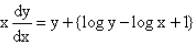is :

Solution:

Given x dy/dx = y(log y - log x+1)
logy-logx=log(y/x)
dy/dx=y/x(log(y/x) +1)
substituting y=vx
dy/dx=v+xdv/dx
=>dv/(vlogv)=dx/x, integrating we get
log(logv)=log(cx)
log(y/x) = cx
=> y/x = e(cx)
=> y = xe(cx)

QUESTION: 5

The solution of the differential equation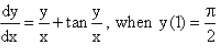is :

Solution:

This is clearly a Homogenous differential equation, as RHS is expressed only in terms of y/x.
To Solve this, Lety/x=t
⟹ y=xt
⟹ dy/dx = t+xdt/dx
By (i)
⟹ tant+t = t+xdt/dx
⟹ tant=xdt/dx
⟹ dx/x=dt/tant
Integrating both sides,
⟹ ∫dx/x = ∫cot tdt
⟹logx = log(sint)+logC
⟹ logx=log(C siny/x)
⟹ x = Csin(y/x)

QUESTION: 6

The solution of the differential equation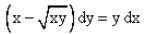is :

Solution:

x -(xy)½ dy = ydx
[x-(xy)½] - y = dx/dy
dx/dy = x/y - [(xy)½]/y……………….(1)
Let V = x/y
x = Vy
dx/dy = V + ydv/dy……..(2)
V + ydv/dy = V - (V)½
ydv/dy = -(V)½
dv/(V)½ = -dy/y
Integrating both the sides, we get
(V(-½+1))/(-½ + 1) = -log y + c
2(V)½ = -log y + c
2(y/x)½ = -log y + c
2(x)½ = (y)½(-log y + c)
2(x)½ = (y)1/2log y + c(y)½
(x)½ = [(y)½]/2 log y + [c(y)½]/2

QUESTION: 7

The solution of the differential equation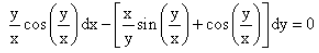is :

Solution:

y/x cos y/x dx − (x/y sin y/x + cosy/x) dy = 0
⇒dy/dx = (y/x cos y/x)/(x/y sin y/x + cos y/x)
This is a homogeneous differential equation.
Putting y = vx and dy/dx = v+xdv/dx, we have v+xdv/dx = (v2cosv)/(sinv+vcosv)
⇒ xdv/dx = (v2cosv)/(sinv+vcosv) - v
⇒x dv/dx = (v2 cosv - v2 cosv - vsinv )/(sinv+vcosv)
⇒ x dv/dx = - [v sinv/(sinv + vcosv)]
⇒ ∫[(sinv + vcosv)/v sinv]dv = ∫dx/x
Integrating both the sides, we get
∫(cot v + 1/v)dv = - ln(x) + c
ln(sin v) + ln(v) = -ln(x) + c
ln(sin(y/x) + ln(y/x) + ln(x) = c
ln(y/x sin y/x * x) + c
y sin(y/x) = c

QUESTION: 8

The solution of the differential equation x2dy - (x2 + 3xy + 4y2) dx = 0 is :

Solution:
QUESTION: 9

The solution of differential equation x2dy + y(x + y)dx = 0 when x = 1, y = 1 is:​

Solution:

x2dy + (xy + y2) dx = 0
⇒ x2 dy = - (xy + y2)dx
⇒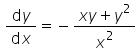.......(i)
Let y = vx
Differentiating w.r.t x we get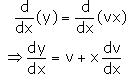Substituting the value of y and dy/dx in equation (1), we get: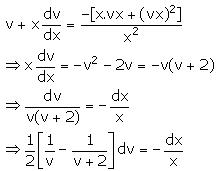Integrating both sides, we get: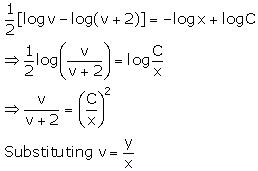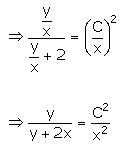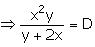......(ii)
Now, it is given that y = 1 at x = 1.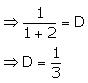Substituting D = 1/3 in equation (2), we get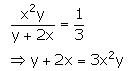QUESTION: 10

The solution of the differential equation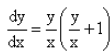is :

Solution: